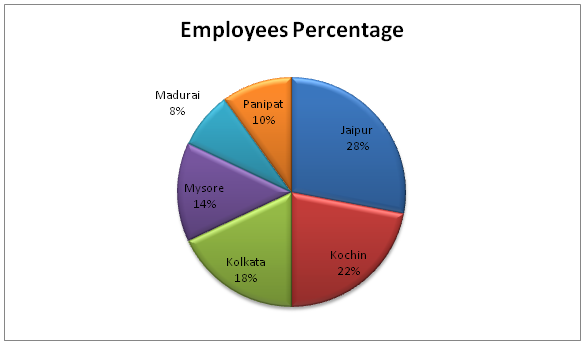# SBI Clerk Prelims 2021 Quantitative Aptitude Questions (Day-57)

Dear Aspirants, Our IBPS Guide team is providing new series of Quantitative Aptitude Questions for SBI Clerk Prelims 2020 so the aspirants can practice it on a daily basis. These questions are framed by our skilled experts after understanding your needs thoroughly. Aspirants can practice these new series questions daily to familiarize with the exact exam pattern and make your preparation effective.

Start Quiz

Approximation

Directions (01-05): What approximate value should come in place of question mark (?) in the following questions?

1) (5/7) of 341 + (2/3) of (7/15) of 1889 =? % of 700

A.150

B.90

C.120

D.210

E.250

2) [48.78 ÷ 7 × 5] ÷ 70 = 18.01 ×?

A.3/47

B.2/35

C.4/57

D.1/36

E.5/74

3) √? + 27.65 = (375.39 + 72.01) ÷ 49.78 + (18.78 × 2.75)

A.1225

B.1444

C.1681

D.961

E.2025

4) 3289 ÷ 14 × 5.01 + 15 % of 500 + 27 % of 299 =?

A.1330

B.1860

C.2150

D.2740

E.1570

5) 36 % of 489.8 + 52 % of 301.25 = 4 ×?

A.75

B.66

C.92

D.127

E.84

Pie chart

Directions (06-10): Study the following information carefully and answer the questions given below.

Following pie chart gives the information about number of employees of Hindustan Company across different cities in percentage.

Total number of Employees in Hindustan company = 22006) If 25% of the total number of employees working in Jaipur is females, how many employees working in Jaipur are male?

A.438

B.462

C.386

D.224

E.None of these

7) If 3/8 of the total number of employees working in Madurai were transferred to Kolkata, how many employees would there be in Kolkata?

A.228

B.396

C.176

D.462

E.None of these

8) Total number of employees working in Mysore is approximately what percent of the total number of employees working in Kochin?

A.46%

B.58%

C.64%

D.36%

E.86%

9) In which company, the total number of employees is more than the total number of employees working in Panipat & Mysore together?

A.Jaipur

C.Kochin

D.Kolkata

E.None of these

10)
What is the respective ratio between the total number of employees working in Kolkata to the total number of employees working in Jaipur?

A.1:5

B.3:7

C.13:15

D.9:14

E.11:13

(5/7) of 343 + (2/3) of (7/15) of 1890 = x % of 700

(5/7) * 343 + (2/3) * (7/15) * 1890 = (x/100)*700

245 + 588 = 7x

833 = 7x

X = 833/7

X = 119 = 120 (Approximately)

[49 ÷ 7 × 5] ÷70 = 18×?

(7*5)/70 = 18x

35/70 = 18x

X = (1/2)*(1/18) = 1/36

√x + 28 = (375 + 72) ÷ 50 + (19 × 3)

√x + 28 = 447/50 + 57

√x = 9 + 57 – 28

√x = 38

X = 382 = 1444

3290 ÷ 14 × 5 + 15 % of 500 + 27 % of 300 = x

(3290/14)*5 + (15/100)*500 + (27/100)*300 = x

X = 1175 + 75 + 81

X = 1331

36 % of 490 + 52 % of 300 = 4 ×?

(36/100)*490 + (52/100)*300 = 4x

4x = 180 + 156

4x = 336

X = (336/4) = 84

X = 84

Total number of employees working in Jaipur = 2200*28/100 = 616

Female employees = 616*25/100 = 154

The male employees working in Jaipur = 616 – 154 = 462

Total no. of employees working in Madurai = 2200*8/100 = 176

Number of employees transferred to Kolkata = 3/8*176 = 66

Then,

Total number of employees in Kolkata = 2200*18/100 + 66

= 396 + 66

= 462

Number of employees working in Mysore = 14/100 * 2200 = 308

Number of employees working in Kochin= 22/100 *2200 = 484

Required percentage = 308/484 *100 = 63.63% = 64% (Approximately)

% of employees working in Panipat&Mysore together = 10% + 14% = 24%

% of employees working in Jaipur = 28 %

% of employees working in Kochin = 22%

% of employees working in Kolkata = 18%

% of employees working in Madurai = 8%

Hence, employees working in Jaipur are more than the employees working in Mysore&Panipat together

Employees working in Kolkata = 18/100 * 2200 = 396

Employees working in Jaipur = 28/100 * 2200 = 616

Required ratio = 396: 616 = 9: 14

Shortcut:

Employees working in Kolkata = 18%

Employees working in Jaipur = 28%

Hence, the required ratio = 18:28 = 9:14

 Check Here to View SBI Clerk Prelims 2021 Quantitative Aptitude Questions Day – 56 Day – 55 Day – 54